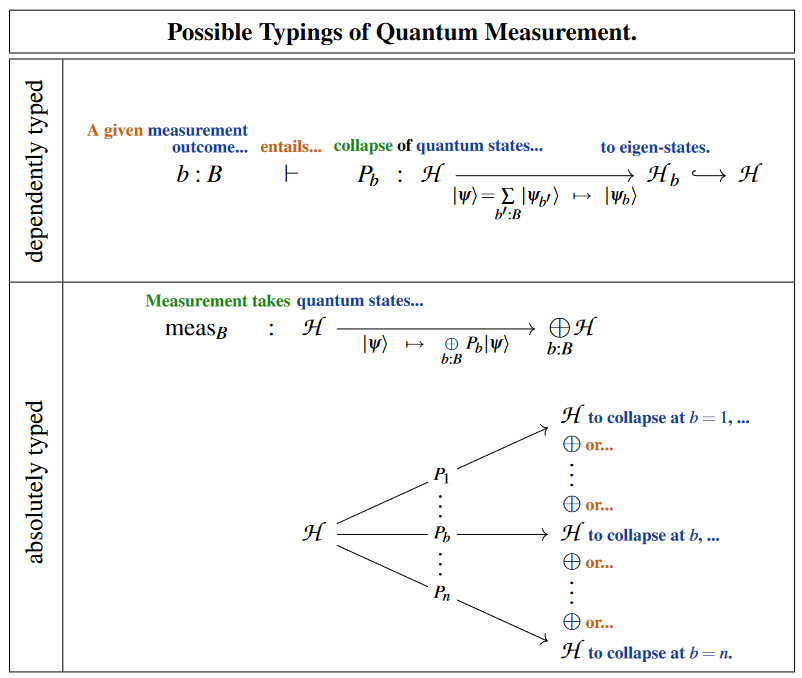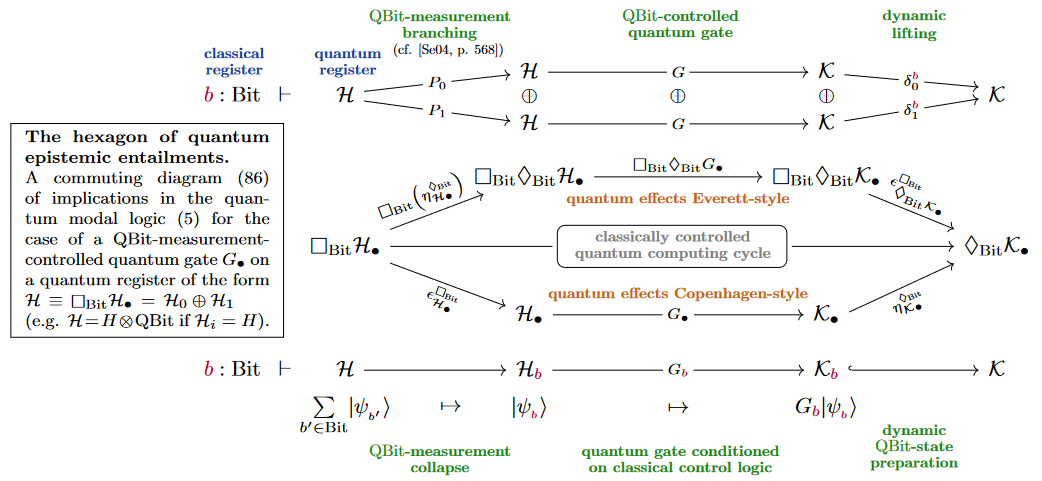# nLab quantum measurement

Quantum measurement

# Quantum measurement

## Idea

Quantum measurement is measurement in quantum mechanics.

The “projection postulate” of quantum physics asserts (von Neumann 1932; Lüders 1951) that:

1. measurement of quantum states is with respect to a choice of orthonormal linear basis $\big\{\vert \psi_b \rangle \big\}_{b : B}$ of the given Hilbert space $\mathscr{H}$ of pure quantum states;

2. the result of measurement on pure quantum states $\vert \psi \rangle \;\in\; \mathscr{H}$ is

1. a random value $b \in B$;

2. the “collapse” of the quantum state being measured by orthogonal projection to the linear span of the $b$th basis state.

$\array{ P_b &\colon& \mathscr{H} &\xrightarrow{\phantom{---}}& \mathscr{H}_b \hookrightarrow \mathscr{H} \\ && \vert \psi \rangle &\mapsto& P_b \vert \psi \rangle \mathrlap{ = \vert \psi_b \rangle \langle \psi_b \vert \psi \rangle } }$

In terms of mixed quantum states represented by density matrices, this prescription translates into a quantum operation which is given by a positive-operator valued measure (this is what Lüders (1951) first wrote down).

There are different ways to type the quantum measurement, taking into account the non-deterministic nature of its outcome:

1. Regarding the direct sum $\bigoplus_{b \colon B} \mathscr{H}$ of Hilbert spaces as the logical disjunction (“or”) of quantum logic, one may regard measurement as being the linear map into the direct sum whose $b$th component is $P_b$.

This choice of typing appears (briefly) in Selinger 2004, p. 39, in a precursor discussion that led to the formulation of the quantum programming language Quipper.

2. Regarding the measurement outcome $b \in B$ as the observed context of the actual quantum collapse, one may regard the collapse projection as dependently typed.

Getting from previous option back to this one is known in the the Quipper-community as dynamic lifting (namely “of the measured bits back into the context”)Both of these options naturally emerge and are naturally unified in the “Quantum Modal Logic” inherent to dependent linear type theory: This is discussed at quantum circuits via dependent linear types.## The “measurement problem”

In the context of interpretation of quantum mechanics it is common to speak of the “measurement problem” when referring to the tension between regarding quantum physics as a probabilistic theory and the idea of realism.

Namely – by the above – a quantum measurement is formally reflected in a change of probabilities. But since in any given measurement experiment one definite outcome is observed, one may wonder how that particular outcome was actually chosen, given that the theory only gives its probability.

(…)

### General

The original axiomatization of quantum measurement via the projection postulate:

Review and discussion:

In view of dynamical quantum decoherence:

Discussion in the refined context of (local algebraic) quantum field theory on Lorentzian manifolds:

### Formalization

Brief mentioning of typing and categorical semantics of quantum measurement

Discussion of quantum measurements in terms of quantum information theory via dagger-compact categories, by Frobenius algebra-structures and the quantum reader monad:

and the evolution of the “classical structures”-monad into the “spider”-diagrams (terminology for special Frobenius normal form, originating in Coecke & Paquette 2008, p. 6, Coecke & Duncan 2008, Thm. 1) of the ZX-calculus:

Textbook account (though without mentioning the monad structure) in:

Generalization to Hilbert bundles:

### The Measurement Problem

The article

points out that for symmetric systems with a symmetric ground state, already a tiny perturbation mixing the ground state with the first excited state causes spontaneous symmetry breaking in a suitable limit, and suggests that this already resolves the measurement problem.